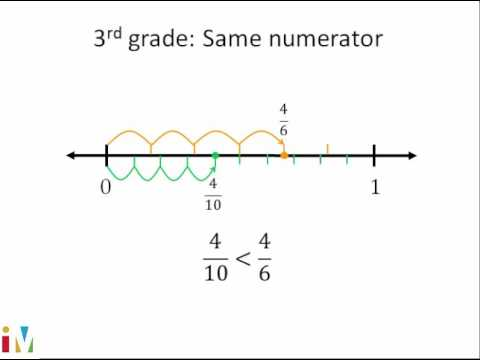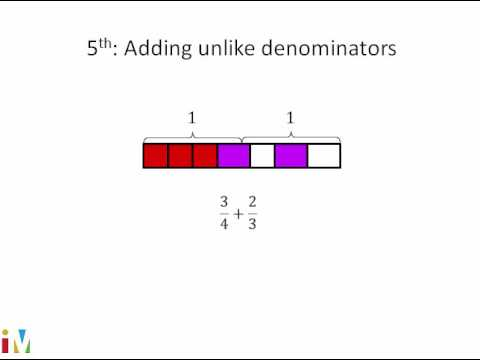COVID-19 presents an unprecedented challenge for teachers, students, and parents. Explore resources from IBM and partners here.
Video

# Multiplying fractions by whole numbers on a number line (Full video)

Description: A short lesson on multiplying fractions by whole numbers on the number line. So let's say we need to figure out, so let's see, it says move the orange dot to the number that equals two times 4/3. Alright, so one times 4/3 is just gonna get us to 4/3, and then if we have another 4/3, we're gonna add 4/3 to that, so we're gonna move another 4/3 to the right. So, move the orange dot to the number that equals three times 3/2.

### Other videos you might be interested in### Comparing Fractions

#### Illustrative Mathematics